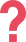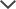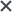## What Is the NWEA 4th Grade MAP Test?

NWEA's 4th Grade MAP Test is an adaptive, computerized test that focuses on the student's abilities in language usage, reading, and math. This exam allows parents and teachers to better understand students' academic needs and areas of growth throughout the school year. A high MAP score can give your fourth grader the opportunity to achieve further academic success.

## 4th Grade MAP Sample Questions

 Question 1: Math What is 694 ÷ 30? A) 21 remainder 4 B) 23 remainder 4 C) 31 D) 31 remainder 3 Answer & Explanation ▼ | ▲ The correct answer is (B). Set up the division:Divide the first two digits by 30: 30 divides into 69 two whole times. It cannot go in three times, because three lots of 30 is 90, which is greater than 69. Write the 2 above the division sum. To find the remainder, subtract the two batches of 30 from the 69: 2 x 30 = 60 // multiply the 30 by the answer 2 and write the result underneath 69 – 60 = 9 // subtract the values to find the remainder.To perform the next division, bring down the remaining 4 to join the 9 and make 94.Now, divide 94 by 30: 30 divides into 94 three whole times. Thus, write the 3 above the division sum. Then, subtract three batches of 30 from 94 to find the remainder. 30 x 3 = 90 94 – 90 = 4Question 2: Math A supermarket packed 60 eggs into four egg boxes, split equally. How many eggs were in three boxes? A) 15 eggs B) 20 eggs C) 36 eggs D) 45 eggs Answer & Explanation ▼ | ▲ The correct answer is (D). This is a question involving rates. There were the same number of eggs packed in each box. If there were 60 eggs in four boxes, then divide 60 by four to find the number of eggs in one box: 60 ÷ 4 = 15 eggs. Thus, there were three boxes each containing 15 eggs. To find the total, either add up 15 + 15 + 15 or calculate 15 x 3 = 45 eggs. Therefore, the correct answer is (D).

 Question 3: Math What is the number 27 in exponential form? A) 2^7 B) 3^3 C) 3^9 D) 9^3 Answer & Explanation ▼ | ▲ The correct answer is (B). Consider each answer in turn: Answer (A) is 2 x 2 x 2 x 2 x 2 x 2 x 2. This will be an even number, as all numbers that are doubled are even and this is a pattern that continues to double. Therefore, it cannot be equal to 27, as 27 is an odd number. Answer (B) is 3 x 3 x 3. First, calculate 3 x 3 = 9. Now, multiply by the next 3: 9 x 3 = 27. This is the correct answer. Continue to check that the rest of the options are incorrect. Answers (C) and (D) are distractors because 3 x 9 = 27. However, in exponent form, these answers are far too high. 39 is much bigger than 33, as it is 33 x 3 x 3 x 3 x 3 x 3 x 3, and thus it cannot be 27. 93 = 9 x 9 x 9. When you multiply the first 9 x 9, you get 81, which is already too big, so there is no need to continue. Answer (E) is 3 x 3 x 3 x 3 x 3, which is also bigger than 27, as it is bigger than 33. Therefore, it cannot be correct.

 Question 4: Language Usage What is the correct spelling for more than one wolf? A) Wolfs B) Wolvs C) Wolves D) Wolfes Answer & Explanation ▼ | ▲ The correct answer is (C). If a singular noun ends in -f and the /f/ sound changes to /v/ when it's plural, drop the -fand add -ves to make the noun plural. One wolf becomes two wolves. Therefore, the correct answer is (C).

 Question 6: Language Usage In which sentence are the words capitalized correctly? A) I was born in the south but grew up in New York. B) If you want to reach the beach, you must keep heading West. C) Turn Right at the end of the street and then Left. D) The new settlers decided to explore the East. Answer & Explanation ▼ | ▲ The correct answer is (D). Directions and the terms that are derived from them (north, east, right, left, etc.) should not be capitalized. Names of regions, geographical locations, and specific places should be capitalized. The only sentence that is capitalized correctly is in answer (D) because the East states a specific location. Therefore, the correct answer is (D). Answer (A) is incorrect because the word "south" should be capitalized as it states a specific region. Answers (B) and (C) are incorrect as directions should not be capitalized.

## 4th Grade MAP Test Scores

MAP tests are graded using the Rasch-Unit scale (RIT). The RIT scale functions as an equal-interval scale that measures each student’s abilities regardless of grade level. This measuring system can be compared to a ruler, in that the difference between each two RIT scores remains the same regardless of whether they are on the top or the bottom of the scale. Ultimately, the purpose of the scale is to provide parents, teachers, and students alike the opportunity to see how much they have progressed academically.

## The MAP Test & The Common Core

Since the use of the Common Core has increased so dramatically in the past few years, NWEA has adjusted every MAP test, including the fourth grade edition, to incorporate its criteria. TestPrep-Online offers practice tests that are adjusted to fit the test's Common Core curriculum. Our NWEA MAP for 4th Grade practice pack covers every component of the Common Core mentioned on the 4th grade MAP Test, making it easier for your child to reach the score he or she deserves.

## NWEA MAP Math Section

The NWEA MAP math section for fourth graders is comprised of four common academic topics that have been taught to the students by the time they have reached the fourth grade.

• Operations and Algebraic Thinking: using the four operations; recognizing patterns; using numerical expressions
• Numbers and Operations: performing mathematical operations and measurements with multi-digit whole numbers, fractions, and decimals
• Measurement and Data: generating, representing, and interpreting data; solving measurement problems using factors such as length, liquid volume, and angle
• Geometry: reasoning and understanding by using geometric concepts; identifying and utilizing three-dimensional figures; solving mathematical problems using graphs

## NWEA MAP Language Usage Section

The three core topics included in the NWEA MAP language usage section are as follows:

• Writing: planning, developing, and revising research and written material
• Grammar and Usage: understanding and implementing grammar conventions
• Understand and Edit Mechanics: understanding and using punctuation, capitalization, and spelling

Generally, the NWEA MAP reading section focuses on informal texts and literature:

• Word Meaning and Vocabulary Knowledge: deciphering word meaning through contextualization; recognizing word relationships and structures
• Literature: analyzing texts, deducing key themes and structure
• Informational texts: analyzing texts, deducing argument and purpose, taking into consideration factors such as point of view and bias

## How to Prepare for the NWEA MAP 4th Grade

To prepare for the 4th Grade MAP test, the golden rule of practice, practice, practice applies. To optimize your fourth grader's practice sessions, practice material should be divided into what is more difficult for the student, and what is easier.

With a variety of practice material to offer, our MAP for 4th grade practice pack is able to help provide a direct reflection of what the student needs during each studying section. Our package includes NWEA replicated practice tests for 4th Grade as well as many sample questions to work with. Your child will not only be able to improve topic-specific knowledge in math, language usage, and reading, but also learn several new topics, all imperative to the test.

The MAP, CogAT, and other trademarks are the property of their respective trademark holders. None of the trademark holders are affiliated with TestPrep-Online or this website.

Need HelpNeed Help